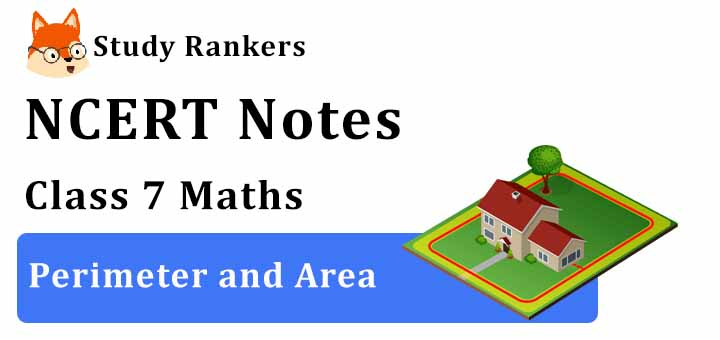>

# Chapter 11 Perimeter and Area Class 7 Notes Maths

Chapter 11 Perimeter and Area Class 7 Notes Maths provided here will provide a quick glimpse of the chapter and improve the learning experience. It will be make sure that a student has understood the specifics of every chapter in clear and precise manner. You will also find NCERT Solutions for Class 7 Chapter 11 Maths which will help you in improving the marks in the examinations and have edge over your classmates. Through these Revision Notes for Class 7, a student can boost their preparation and assessment of understood concepts.Squares and Rectangles

• Perimeter of a regular polygon = number of sides × length of one side

• Perimeter of a square = 4 × side

• Perimeter of a rectangle = 2 × (l + b)

• Area of a rectangle = l × b

• Area of a square = side × side

Triangles as Parts of Rectangles

• If we cut the rectangle along its diagonal to get two triangles, then
The area of each triangle = 1/2 (Area of the rectangle)

• If we take a square and divide it into 4 triangles, then
The area of each triangle = 1/4 (Area of the square)

Area of Parallelogram

• The area of parallelogram = base × height.

• Any side of a parallelogram can be chosen as base of the parallelogram. The perpendicular dropped on that side from the opposite vertex is known as height (altitude).

Area of a Triangle

• The area of triangle = 1/2 × base × height.

• Are of equilateral triangle = √3/4 × (side)2

• All the congruent triangles are equal in area but the triangles equal in area need not be congruent.

Circle

• The distance around a circle is known as its circumference.

• The ratio of circumference and diameter of a circle is a constant and is denoted by it (pi).

• Approximate value of π is taken as 22/7 or 3.14.

• Circumference of a circle of radius r = 2πr

• Area of a circle of radius r = πr2.